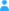Sign in

|

## Primary data

### Releases

Information about all releases of the current dataset #4513 and their availability. The release number is automatically incremented by 1 for each update, starting at 1 for the first release.

### Further Information

• Begin of free primary data access: in 76 years and 3 months*

## Entries (observed variables)

Information about observed variables of the current dataset.
No. Name
A virtual entry: n_height given by 44 constant values
B virtual entry: n_cublen given by 15 constant values
C virtual entry: n_prof given by 984 constant values
01 Internal name for measurement file of interferogram.
02 Time UTC (seconds since 2000-1-1 0:0:0)
03 Latitude [degree_north]
04 Longitude [degree_east]
05 Altitude of observer/aircraft (km)
06 viewing direction of observer [degree]
07 Altitude (km)
08 air_​temperature [unspecified unit]
09 Total estimated error of temperature volume mixing ratio (K)
10 Precision of temperature volume mixing ratio (K)
11 Accuracy of temperature volume mixing ratio (K)
12 Retrieval noise of temperature volume mixing ratio (K)
13 Vertical resolution of temperature volume mixing ratio (km)
14 Initial guess profiles of temperature volume mixing ratio (K)
15 Diagonal element of averaging kernel of temperature volume mixing ratio 
16 mole_​fraction_​of_​nitric_​acid_​in_​air [unspecified unit]
17 Total estimated error of HNO3 volume mixing ratio (ppmv)
18 Precision of HNO3 volume mixing ratio (ppmv)
19 Accuracy of HNO3 volume mixing ratio (ppmv)
20 Retrieval noise of HNO3 volume mixing ratio (ppmv)
21 Vertical resolution of HNO3 volume mixing ratio (km)
22 Initial guess profiles of HNO3 volume mixing ratio (ppmv)
23 Diagonal element of averaging kernel of HNO3 volume mixing ratio 
24 mole_​fraction_​of_​ozone_​in_​air [unspecified unit]
25 Total estimated error of O3 volume mixing ratio (ppmv)
26 Precision of O3 volume mixing ratio (ppmv)
27 Accuracy of O3 volume mixing ratio (ppmv)
28 Retrieval noise of O3 volume mixing ratio (ppmv)
29 Vertical resolution of O3 volume mixing ratio (km)
30 Initial guess profiles of O3 volume mixing ratio (ppmv)
31 Diagonal element of averaging kernel of O3 volume mixing ratio 
32 mole_​fraction_​of_​cfc12_​in_​air [unspecified unit]
33 Total estimated error of CFC-12 volume mixing ratio (ppmv)
34 Precision of CFC-12 volume mixing ratio (ppmv)
35 Accuracy of CFC-12 volume mixing ratio (ppmv)
36 Retrieval noise of CFC-12 volume mixing ratio (ppmv)
37 Vertical resolution of CFC-12 volume mixing ratio (km)
38 Initial guess profiles of CFC-12 volume mixing ratio (ppmv)
39 Diagonal element of averaging kernel of CFC-12 volume mixing ratio 
40 mole_​fraction_​of_​chlorine_​nitrate_​in_​air [unspecified unit]
41 Total estimated error of ClONO2 volume mixing ratio (ppmv)
42 Precision of ClONO2 volume mixing ratio (ppmv)
43 Accuracy of ClONO2 volume mixing ratio (ppmv)
44 Retrieval noise of ClONO2 volume mixing ratio (ppmv)
45 Vertical resolution of ClONO2 volume mixing ratio (km)
46 Initial guess profiles of ClONO2 volume mixing ratio (ppmv)
47 Diagonal element of averaging kernel of ClONO2 volume mixing ratio 
48 mole_​fraction_​of_​cfc11_​in_​air [unspecified unit]
49 Total estimated error of CFC-11 volume mixing ratio (ppmv)
50 Precision of CFC-11 volume mixing ratio (ppmv)
51 Accuracy of CFC-11 volume mixing ratio (ppmv)
52 Retrieval noise of CFC-11 volume mixing ratio (ppmv)
53 Vertical resolution of CFC-11 volume mixing ratio (km)
54 Initial guess profiles of CFC-11 volume mixing ratio (ppmv)
55 Diagonal element of averaging kernel of CFC-11 volume mixing ratio 
56 mole_fraction_of_water_vapour_in_air (ppmv)
57 Total estimated error of H2O volume mixing ratio (ppmv)
58 Precision of H2O volume mixing ratio (ppmv)
59 Accuracy of H2O volume mixing ratio (ppmv)
60 Retrieval noise of H2O volume mixing ratio (ppmv)
61 Vertical resolution of H2O volume mixing ratio (km)
62 Initial guess profiles of H2O volume mixing ratio (ppmv)
63 Diagonal element of averaging kernel of H2O volume mixing ratio 
64 mole_​fraction_​of_​ethyne_​in_​air [unspecified unit]
65 Retrieval noise of C2H2 volume mixing ratio (ppmv)
66 Vertical resolution of C2H2 volume mixing ratio (km)
67 Initial guess profiles of C2H2 volume mixing ratio (ppmv)
68 Diagonal element of averaging kernel of C2H2 volume mixing ratio 
69 mole_​fraction_​of_​ethane_​in_​air [unspecified unit]
70 Retrieval noise of C2H6 volume mixing ratio (ppmv)
71 Vertical resolution of C2H6 volume mixing ratio (km)
72 Initial guess profiles of C2H6 volume mixing ratio (ppmv)
73 Diagonal element of averaging kernel of C2H6 volume mixing ratio 
74 mole_​fraction_​of_​peroxyacetyl_​nitrate_​in_​air [unspecified unit]
75 Retrieval noise of PAN volume mixing ratio (ppmv)
76 Vertical resolution of PAN volume mixing ratio (km)
77 Initial guess profiles of PAN volume mixing ratio (ppmv)
78 Diagonal element of averaging kernel of PAN volume mixing ratio 
79 mole_fraction_of_formic_acid_in_air (ppmv)
80 Retrieval noise of HCOOH volume mixing ratio (ppmv)
81 Vertical resolution of HCOOH volume mixing ratio (km)
82 Initial guess profiles of HCOOH volume mixing ratio (ppmv)
83 Diagonal element of averaging kernel of HCOOH volume mixing ratio 
84 air_​pressure [unspecified unit]
85 ertel_​potential_​vorticity [unspecified unit]
86 Cloud Index (lower values -> more clouds!) interpolated on GLORIA geolocations 
87 air_​potential_​temperature [unspecified unit]
88 ECMWF equivalent latitude interpolated on GLORIA geolocations (K)

To browse for the dependencies of the observed variables, please use this link.

## Dataset Info

Name of dataset

Short:
#4513
Full:
#4513 | F12_20160131_GLORIA_chemistry_mode_KIT.nc

Date last revised

2020-05-06

Time period of the primary data

Begin:
2015-12-07*
End:
2016-03-21*

* inherited from the metadata of the mission POLSTRACC

Data format

## Data Origin

Genesis of the data (see glossary):

Ongoing Subset (see glossary):

160131a